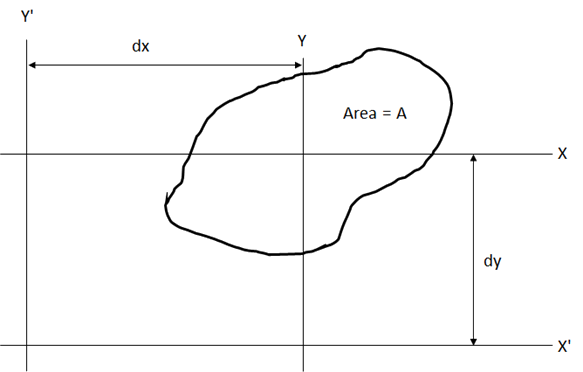Reference: Abbott, Richard. Analysis and Design of Composite and Metallic Flight Vehicle Structures 3 Edition, 2019

If the moment of inertia of a cross section about a centroidal axis is known, then the parallel axis theorem can be used to calculate the moment of inertia about any parallel axis:Figure 6.1.4‑1: Definition of terms for Parallel Axis Theory